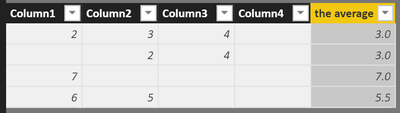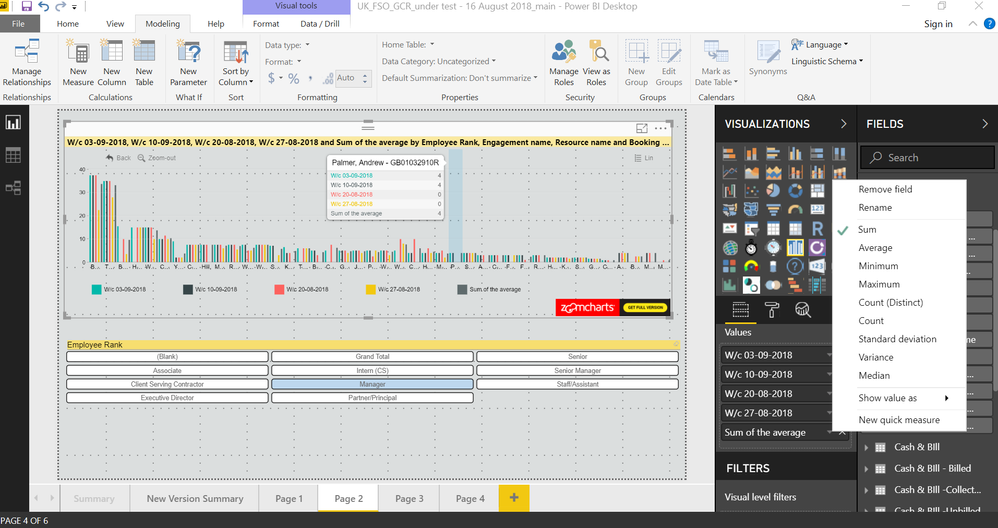cancel
Showing results for
Did you mean:Anonymous
Not applicable

## How to calculate average value with null values

My query is related to handling null values in calculating the average at row level. Suppose we have 4 columns.
COL1 COL2 COL3 COL4
11 12 11 NULL
24 22 NULL 33
44 NULL NULL 44
55 NULL NULL 55
My query is suppose we need to get average of all the 4 columns i.e. (COL1+COL2+COL3+COL4)/4. As some of column contains null value so we cannot use above method for finding the average i.e. col1+col2.... Also we cannot replace NULL with 0 using ISNULL() as it reduces the average value, in short we need to take only those columns in count which has value and need to hide null values i.e. in first row avg should be of (col1+col2+col3)/3.
Please let me know if anyone has faced this scenario in past and what solution he/she opted?

1 ACCEPTED SOLUTIONSuper User

Hey,

I used this DAX statement to create a "calculated column"

```the average =
var theTable =
UNION(
ROW("value",'Table1'[Column1])
,ROW("value",'Table1'[Column2])
,ROW("value",'Table1'[Column3])
,ROW("value",'Table1'[Column4])
)
var theSum = 'Table1'[Column1] + 'Table1'[Column2] + 'Table1'[Column3] + 'Table1'[Column4]
var theDivisor =
COUNTROWS(
FILTER(
theTable
,[value] <> BLANK()
)
)
return DIVIDE(theSum, theDivisor, BLANK())```

All the columns have a numeric data tape like decimal.

I create a table from the columns that have to be considered using UNION(ROW(...),...)

I create a simple sum from the values of in the columns that have to be considered

I count the non empty rows in the table.

Based on my sample data this will retrun these results:Hopefully this is what you are looking for,

Regards,

Tom

Did I answer your question? Mark my post as a solution, this will help others!

Proud to be a Super User!
I accept Kudos 😉
Hamburg, Germany
4 REPLIES 4Super User

Hey,

I used this DAX statement to create a "calculated column"

```the average =
var theTable =
UNION(
ROW("value",'Table1'[Column1])
,ROW("value",'Table1'[Column2])
,ROW("value",'Table1'[Column3])
,ROW("value",'Table1'[Column4])
)
var theSum = 'Table1'[Column1] + 'Table1'[Column2] + 'Table1'[Column3] + 'Table1'[Column4]
var theDivisor =
COUNTROWS(
FILTER(
theTable
,[value] <> BLANK()
)
)
return DIVIDE(theSum, theDivisor, BLANK())```

All the columns have a numeric data tape like decimal.

I create a table from the columns that have to be considered using UNION(ROW(...),...)

I create a simple sum from the values of in the columns that have to be considered

I count the non empty rows in the table.

Based on my sample data this will retrun these results:Hopefully this is what you are looking for,

Regards,

Tom

Did I answer your question? Mark my post as a solution, this will help others!

Proud to be a Super User!
I accept Kudos 😉
Hamburg, GermanyAnonymous
Not applicable

Hello Tom

How can I apply that solution when i have a unique colum, with some nulls values, and I need the average (as a metric) of that unique colum? ThanksAnonymous
Not applicable

Hi @TomMartens,

Greetings !!

Excellent it is working well now. Thanks a lot.Anonymous
Not applicable

Hi,

when i added "the average" calculated column into report, i validated data and feel it is coming incorrectly bcoz of getting disable of Don't summarize option.

If you screenshot attached, will understand clearly. Thanks.Announcements#### Exclusive opportunity for Women!

Join us for a free, hands-on Microsoft workshop led by women trainers for women where you will learn how to build a Dashboard in a Day!#### Power Platform Conference-Power BI and Fabric Sessions

Join us Oct 1 - 6 in Las Vegas for the Microsoft Power Platform Conference.Top Solution Authors
Top Kudoed Authors
Users online (3,786)